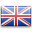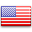## Math

Refreshe Todd
Posts: 1
Joined: Fri Nov 18, 2022 4:37 pm
Gender:I'm having quite a bit of trouble with algebraic equations.
For example, Spongebob gave some homework the other day and the equation was: y=-1x+12
and to graph it.
I would appreciate some help.
Thanks!
Crispy
Posts: 1
Joined: Thu Nov 24, 2022 1:30 am
Gender:Hey Todd,

Algebra can be fun to do once you get the hang of it. If we take your equation:

y=-1x+12

You'll want to isolate the x, meaning, you want a positive x all by itself on one side of the equal sign (for example: x=45)

Usually, a -x or x is presented by only the x, not a 1x, but they both mean the same thing. You also have to remember that 12 and -1x are different coefficients, so you can't add -1 and 12 because -1x is not a constant like 12 is (hint: if it has a different ending [ie. x, x^2, y, etc.] you can only combine them with themselves). So, let's solve now.

y=-1x+12

You must first move the 12 to the other side of the equation to make

y=-1x+12
-12 -12

-12=-1x

After, we isolate the x by dividing by -1 because -1x represents -1*x, and when we want to get rid of a multiplier, we divide and vice versa. And remember, dividing or multiplying 2 negatives together results in a positive number. So now we have

-12=-1x
--------------
-1

12=x

So now, our answer is 12=x. Another helpful tip I will give is whenever you are isolating the x for an inequality equation, always remember to flip the greater than/less than sign whenever you divide by a negative number.

Have a good day.